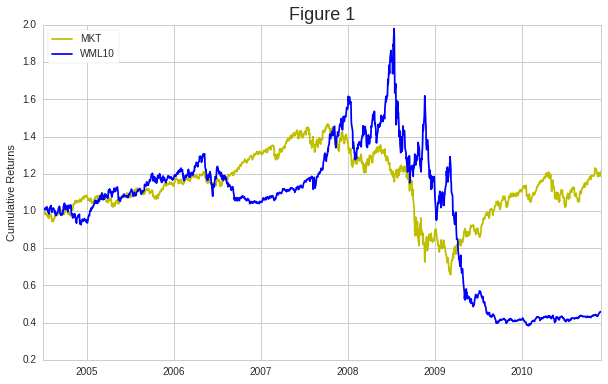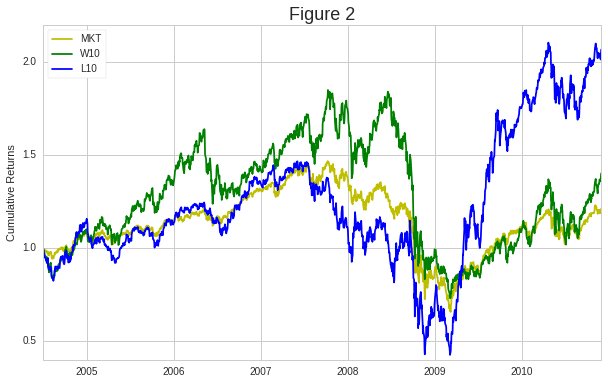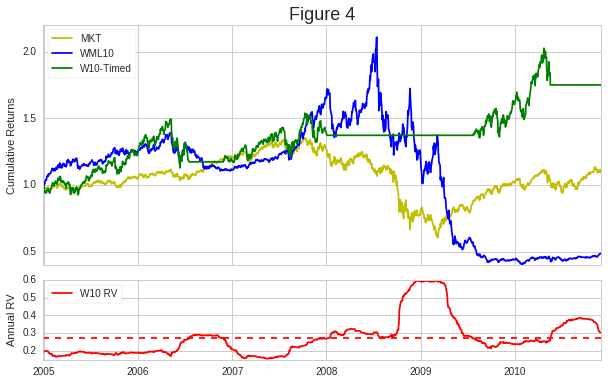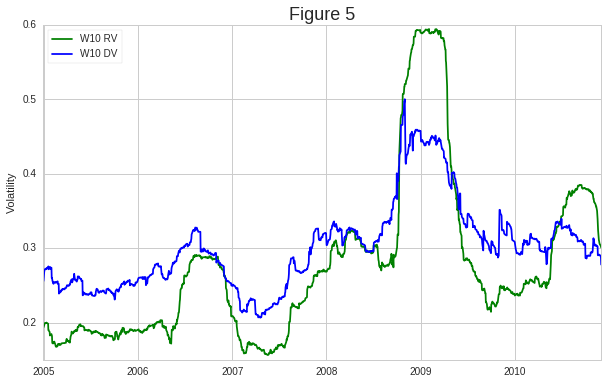Notebook

This notebook features the Quantopian-based research presented in the paper "Momentum with Volatility Timing". Specifically, the paper proposes two extensions to the conventional momentum factor strategy by introducing the volatility-timed winners approach. First, for resolving factor underperformance in the 2011-2018 post-crisis period, the conventional winners-minus-losers momentum is replaced with the winners-only component. Second, the proposed approach substitutes constant volatility scaling with the threshold function and uses past volatilities as a timing predictor for changing momentum strategies. The approach was confirmed with Spearman rank correlation and demonstrated in relation to different strategies including momentum volatility scaling, risk-based asset allocation, time series momentum and MSCI momentum indexes.

The notebook is structured as follows:

In :
import datetime
import numpy as np
import pandas as pd
from pandas.tseries.offsets import BDay
import matplotlib.pyplot as plt
from quantopian.pipeline import Pipeline
from quantopian.pipeline.data.builtin import USEquityPricing
from quantopian.pipeline.factors import CustomFactor, Returns, DailyReturns
from quantopian.research import run_pipeline
import alphalens as al
import math

In :
import pyfolio as pf
import empyrical as ep


## 1. Quantopian and Alphalens Processing¶

This section is based on the Quantopian and Alphalens framework that is comprehensively described in Lecture 39: Factor Analysis with Alphalens. In comparisson with the lecture, however, the paper applies the Quantopian framework to the conventional winners-minus-losers momentum strategy that was documented by Jegadeesh and Titman (1993). The corresponding factor is computed by ranking stocks according to their prior behavior over the course of 11 months with a 1 month lag:

In :
class Momentum(CustomFactor):
""" Conventional Momentum factor """
inputs = [USEquityPricing.close]
window_length = 252

def compute(self, today, assets, out, prices):
out[:] = (prices[-21] - prices[-252])/prices[-252]


Defining time interval covering the 2008-2009 market downturn

In :
start_date, end_date = '2004-07-01', '2010-12-31'


Running pipeline with Momentum class for calculating momentum prior scores

In :
universe = QTradableStocksUS()

def make_pipeline():

pipe = Pipeline()
pipe.set_screen(universe)

return pipe

pipe = make_pipeline()
results = run_pipeline(pipe, start_date, end_date)
results.dropna(inplace=True)

mom_scores = results['momentum']


Pipeline Execution Time: 20.83 Seconds

Retrieving prices for pipeline assets

In :
asset_list = results.index.levels
prices = get_pricing(asset_list, start_date=start_date, end_date=end_date, fields='open_price')


Calculating forward returns and quantile ranks with Alphalens

In :
mom_data = al.utils.get_clean_factor_and_forward_returns(factor=mom_scores,
prices=prices,
quantiles=10,
periods=(1, 21))

Dropped 1.5% entries from factor data: 1.5% in forward returns computation and 0.0% in binning phase (set max_loss=0 to see potentially suppressed Exceptions).
max_loss is 35.0%, not exceeded: OK!


Computing returns for factor quantiles

In :
mom_returns, mom_stds = al.performance.mean_return_by_quantile(mom_data,
by_date=True,
by_group=False,
demeaned=False,
mom_returns_1D = mom_returns['1D'].unstack('factor_quantile')


## 2. Winners-Minus-Losers Momentum Versus Market¶

The section compares the performance of the conventional winners-minus-losers (WML) momentum strategy and market. As shown in Figure 1, during the recession the market cumulative returns began to steeply fall and reached a low in 2009. Afterwards, the economy began to recover and the returns subsequently started increasing. The WML momentum strategy, in contrast, fared well through the recession, with returns soaring through the duration of the 2008-2009 economic downturn, but the factor then experienced an abrupt, substantial momentum crash the moment excess market returns began to recover. Momentum’s performance subsequently fell below its 2005 level and finished 2010 below the market.

WML is calculated by grouping the top and bottom 10% of assets into equally-weighted winners and losers portfolios, respectively, and then taking the difference of these components.

Note: The momentum factors in the Carhart factor model and the Kenneth R. French Data Library are computed using the top and bottom 30% of assets.

In :
wml10 = mom_returns_1D - mom_returns_1D


Retrieving market prices and returns with the SPDR S&P 500 ETF Trust SPY index

Note: In the paper, the risk-free rate is subtracted from the market, winners, and losers returns. The BIL risk-free proxy available through get_pricing, however, goes back only to May 2007. Therefore, as an alternative the risk-free rates from the Kenneth R. French Data Library can be uploaded to a notebook and applied. For simplicity this notebook ignores RF.

In :
mkt_start_date = datetime.datetime.strptime(start_date, '%Y-%m-%d') - BDay(1)
mkt_prices = get_pricing('SPY', start_date=mkt_start_date, end_date=end_date, fields='price')
mkt_returns = mkt_prices.pct_change(periods=1)[1:-21]


Calculating cumulative returns and comparing performance

In :
days = wml10.index.values
mkt_cum = np.cumprod(mkt_returns + 1)
wml10_cum = np.cumprod(wml10 + 1)

plt.figure(figsize=(10,6))
plt.plot(days, mkt_cum/mkt_cum, color='y')
plt.plot(days, wml10_cum/wml10_cum, color='blue')
plt.title('Figure 1', fontsize=18)
plt.ylabel('Cumulative Returns')
plt.legend(['MKT','WML10'],loc='upper left')
plt.show()## 3. Assessment of Momentum Underperformance with Winners and Losers Components¶

To gain insight into the behavior of WML10, we need to extend the analysis towards the consideration of the factor’s winning and losing components, W10 and L10, respectively. According to Figure 2, the spike in WML10 cumulative returns in mid-2008 was caused by L10 shifting downwards, resulting in a larger gap between winners and losers. Then, after the market began to regain strength and subsequently momentum crashed, the top and bottom 10% portfolios switched places: losers outperformed winners. Therefore, the momentum winners-minus-losers strategy became no longer profitable and resulted in going long the underperforming past winners while shorting overperforming past losers.

In :
w10 = mom_returns_1D
w10_cum = np.cumprod(w10 + 1)
l10 = mom_returns_1D
l10_cum = np.cumprod(l10 + 1)

plt.figure(figsize=(10,6))
plt.plot(days, mkt_cum/mkt_cum, color='y')
plt.plot(days, w10_cum/w10_cum, color='green')
plt.plot(days, l10_cum/l10_cum, color='blue')
plt.title('Figure 2', fontsize=18)
plt.ylabel('Cumulative Returns')
plt.legend(['MKT','W10','L10'],loc='upper left')
plt.show()## 4. Volatility-Timed Winners Approach¶

The momentum underperformance during the market downturn is addressed by different volatility-scaling approaches (e.g., Moskowitz, Ooi, Pedersen, 2012; Barroso and Santa-Clara, 2015). As will be shown, the market downturn broke the conceptual correlation between priors and forward returns. Therefore, the paper proposed to bypass this interval using volatility as a timing predictor and replacing scaling with the threshold function.

Defining the time window for calculating realized volatility

In :
vw = 126


Calculating realized volatility and volatility-timed winners

In :
w10_r2 = w10**2
w10_rv = np.sqrt(w10_r2.rolling(vw).sum()*2)

w10_scale = 0.27/w10_rv
w10_thrd = w10_scale.apply(lambda x: np.where(x < 1, 0, 1))
w10_timed = w10*w10_thrd


Comparing performance of the volatility-timed winners approach and the conventional winners-minus-losers momentum

In :
w10_timed_cum = np.cumprod(w10_timed[vw:]+1)

f, (ax1, ax2) = plt.subplots(2, sharex=True, sharey=False, gridspec_kw={'height_ratios': [3, 1]}, figsize=(10,6))

#Cumulative returns
ax1.plot(days[vw:], mkt_cum[vw:]/mkt_cum[vw], color='y', label="MKT")
ax1.plot(days[vw:], wml10_cum[vw:]/wml10_cum[vw], color="blue", label="WML10")
ax1.plot(days[vw:], w10_timed_cum/w10_timed_cum, color="green", label="W10-Timed")
ax1.set_ylabel('Cumulative Returns')
ax1.set_title('Figure 4', fontsize=18)
ax1.legend(loc='upper left')

#Annualized realized volatility
ax2.plot(days[vw:], w10_rv[vw:], color="red", label="W10 RV")
ax2.set_ylabel('Annual RV')
ax2.axhline(y=0.27, color='red', linestyle='--')
ax2.legend(loc='upper left')## 5. Spearman Rank Correlation Analysis¶

The volatility-timed approach highlighted the relationship between the performance of the momentum factor and market downturn. Therefore, the momentum strategy was further investigated with Spearman rank correlation described in Lecture 23. The coefficients were computed between the 11 month momentum factor prior and 21-day forward returns. According to Table 1, this correlation during the 2005-2010 interval had a p-value of 0.6 and failed to reject the null hypothesis at alpha 1% of no monotonic relationship between the ranked variables. Then, as shown in Table 2, the volatility-timed winners approach resolved and enhanced the rank correlation between the momentum prior and forward returns by capturing and excluding the interval with the negative oscillations.

Table 1: Conventional Momentum Information Coefficient

In :
ic = al.performance.factor_information_coefficient(mom_data)
al.plotting.plot_information_table(ic[vw:])

Information Analysis

1D 21D
IC Mean 0.009 -0.003
IC Std. 0.149 0.173
t-stat(IC) 2.294 -0.568
p-value(IC) 0.022 0.570
IC Skew -0.202 -0.730
IC Kurtosis 0.287 0.747

Removing the volatility-timed intervals from the momentum data

In :
w10_rv_timed = w10_rv[vw:][w10_rv < 0.27]
ts= [pd.Timestamp(x).tz_localize('UTC') for x in w10_rv_timed.index.values]
mom_data_timed = mom_data.loc[ts]


Table 2: Volatility-Based Information Coefficient

In :
ic_timed = al.performance.factor_information_coefficient(mom_data_timed)
al.plotting.plot_information_table(ic_timed)

Information Analysis

1D 21D
IC Mean 0.015 0.030
IC Std. 0.126 0.133
t-stat(IC) 3.444 6.586
p-value(IC) 0.001 0.000
IC Skew -0.291 -0.615
IC Kurtosis 0.119 0.757

## 6. IDE-Oriented Pipeline¶

The section shows a pipeline implemented from the IDE-based variant suggested by @Vladimir within this thread. In brief, the pipeline from Section 1 was extended with DownsideVolatility and PercentileFilter which replaced Alphalens-based factor processing.

In :
class DownsideVolatility(CustomFactor):
inputs = [DailyReturns()]
window_length = 126

def compute(self, today, assets, out, returns):
returns[returns > 0] = np.nan
down_vol = np.nanstd(returns, axis = 0)
ann_down_vol = down_vol*math.sqrt(252)
out[:] = ann_down_vol

class RV(CustomFactor):
inputs = [DailyReturns()]
window_length = 1
r2s = np.zeros(126)

def compute(self, today, assets, out, returns):
r = np.nanmean(returns)
r2 = r**2
self.r2s[:-1] = self.r2s[1:]; self.r2s[-1] = r2
rv = np.sqrt(np.sum(self.r2s)*2)
out[:] = np.ones(returns.shape)*rv

def make_pipeline():

pipe = Pipeline()

mom_w = mom.winsorize (0.01, 1. - 0.01)
w10_filter = mom_w.percentile_between(90, 100)

pipe.set_screen(universe)

return pipe

w10_pipeline = make_pipeline()
results = run_pipeline(w10_pipeline, start_date, end_date)
results.dropna(inplace=True)


Pipeline Execution Time: 15.34 Seconds

Comparing downside volatility with the momentum realized volatility from Section 4. The former is related to the asset-oriented time series momentum approach described in the Comparison with Time Series Momentum and Risk-Based Allocation section (see Eq. 4 and Eq. 9). According to Figure 5 (and Exhibit 9), the corresponding volatilities require different thresholds.

In :
w10_dv = results['dv'].mean(level=0)

plt.figure(figsize=(10,6))
plt.plot(days[vw:], w10_rv[vw:], color = 'g')
plt.plot(days[vw:], w10_dv[vw:-21], color = 'b')
plt.title('Figure 5', fontsize=18)
plt.ylabel('Volatility')
plt.legend(['W10 RV','W10 DV'], loc = 'upper left')
plt.show()## 7. Pyfolio Analysis¶

The section presents the summary statistics and drawdown periods of the Pyfolio tearsheet (see Lecture 33: Portfolio Analysis)

In :
pf.plotting.show_perf_stats(w10_timed[vw:], mkt_returns[vw:])

Start date 2004-12-30 2010-12-01 71 9.9% 74.6% 17.5% 0.63 0.40 0.77 -24.5% 1.15 0.88 -0.31 4.08 0.96 -2.2% 0.10 0.23
In :
pf.plotting.show_worst_drawdown_periods(w10_timed[vw:],5)

Worst drawdown periods Net drawdown in % Peak date Valley date Recovery date Duration
0 24.48 2006-05-09 2006-06-13 2007-10-08 370
1 14.18 2007-10-10 2007-11-20 2009-10-09 523
2 13.59 2010-04-23 2010-05-18 NaT NaN
3 13.47 2009-10-19 2009-11-02 2009-12-21 46
4 12.94 2005-03-04 2005-05-13 2005-06-16 75